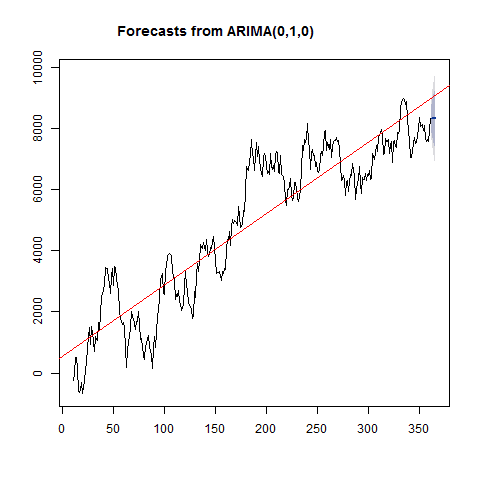### Is the stock market due for a correction?

7/03/2014 05:19:00 PM
Tweetable
Does it even make sense to ask?

A friend of mine is worried that the stock market might have a "correction" soon, and is thinking about selling off his stocks in anticipation. Looking at the Dow Jones Industrial Average (DJIA). It's easy to see where this talk is coming from:Graph of the Dow Jones Industrial Average, monthly, January 1985 to present.From this graph, it appears that we are currently well above the historical trend, and therefore due for a correction. On twitter, Seth Trueger said what I think most people are thinking:
Indeed, if there is a tendency for the stock market to regress to the mean ("mean," in this case, meaning the trend line in red), then the fact that we are currently above the trend line implies we should expect a higher than average probability of a downward movement in the stock market--that is, a "correction" that returns us to the true, underlying time-trend. We'll call this the One True Trend theory of the stock market.

But time series are much trickier than that. Series that don't have regression towards the mean can look quite a bit like series that do. Consider, for example, a random walk with drift:$$y_t=y_{t-1}+\alpha+\epsilon_t,$$where $y_t$ is this month's stock index, $y_{t-1}$ is last month's index value (a coefficient of 1 means this is a random walk), $\alpha$ is a constant (the "drift" term), and $\epsilon_t$ is this month's random shock, which is normally distributed with mean zero. This process will produce a graph that looks a lot like the stock market with a clear, unambiguous time trend caused by the drift term, and will appear prima facie to exhibit reversion to the mean, because the error term is symmetric--negative shocks are as common and as large as positive shocks, so the index will appear to bounce around a common time trend, on average. But there actually is no One True Trend in this process--all shocks are permanent, being above trend in one period has absolutely no effect on the probability of the index rising or falling in the next period. If, on the other hand, the coefficient on $y_{t-1}$ is less than 1, then the impact of random shocks would diminish over time, resulting in reversion to the mean--maintaining an above-trend index value would require a sequence of increasingly extreme, and thus decreasingly likely, random shocks. That is, when shocks are transitory rather than permanent, being above trend increases the likelihood of a decrease in the stock price--a "correction," if you will.

The question above is just a restatement of what is more formally known as the unit root hypothesis. The autoregressive process above, with a coefficient of 1
on $y_{t-1}$, has a unit root, which implies that each realization of $\epsilon_t$ actually shifts the entire time-trend permanently. With a unit root, there is no One True Trend--the observed time trend is actually variable, dependent on the entire history of realizations of $\epsilon_t$ since the beginning of time. And since the next realization will also shift the time trend permanently, whether we are above or below "trend" cannot possibly predict the future direction of the index, ever. It turns out that the (stationary) ARIMA model that minimizes the Akaike information criterion (in a sense, the best-fitting valid model) is an ARIMA(0,1,0) with drift. That corresponds to exactly the equation above, and yes, it has a unit root:$$y_t=y_{t-1}+43.7+\epsilon_t.$$Sorry folks, but as best we can tell from this one series, the One True Trend is apocryphal, and there is no such thing as a "correction." What we perceive as corrections are actually just totally random shocks. I've plotted the forecast below, and as you can see it does not predict a "correction." In fact, an increase in the DJIA remains slightly more likely than a decrease, despite the fact that we are way above the apparent trend:Forecast of the DJIA

To drive home the point, I did a few simulations calibrated to the exact ARIMA process estimated above. Out of slightly more than 100 simulations, here are a few that looked interesting:Prima facie, this graph would appear to suggesst a tendency for reversion to the mean. But if you're predicting a future of rapid growth because we're below trend, you'd be quite mistaken.This looks a lot like the stock market, and many other macro time series. But as in the other simulations, being currently below trend conveys no information whatsoever.Nope, this graph does not imply we are in the midst of a massive bubble.While the graph strongly suggests a tendency to return to trend, this is actually not true of the process that generated it.
As these simulations show, random walks with drift often do seem to bounce around a One True Trend, but this is entirely an illusion: these are all simulations from a process in which distance from trend contains absolutely no information about the likely direction of future stock movements.

But then again, I did mention that time series are tricky. We must avoid committing the Mankiw Unit Root Fallacy--the fact that our data is empirically consistent with a unit root does not actually imply that all shocks are necessarily permanent and inevitable. A bivariate analysis may detect a combination of transitory and permanent shocks and, either way, there's no reason to suppose that policies can't affect stock values, or that insider information isn't predictive of stock prices. But, what I can say is that if you are one of the many people worrying that stock prices are above trend, stop--your fears are empirically unfounded. Perhaps Bill Gardner said it best:Indeed. Empirically, the history of stock prices adds nothing to your priors.

As always, you can review my data and script file.